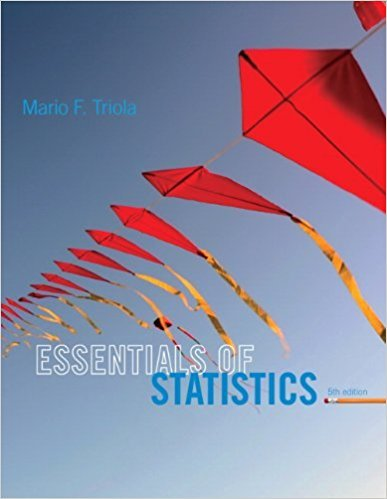×
×

# Statistical Significance and Practical | Ch 1 - 7ISBN: 9780321924599 237

## Solution for problem 7 Chapter 1

Essentials of Statistics | 5th Edition

• Textbook Solutions
• 2901 Step-by-step solutions solved by professors and subject experts
• Get 24/7 help from StudySoup virtual teaching assistantsEssentials of Statistics | 5th Edition

4 5 1 419 Reviews
20
4
Problem 7

Statistical Significance and Practical Significance The Gengene Research Group hasdeveloped a procedure designed to increase the likelihood that a baby will be born a girl. In aclinical trial of their procedure, 112 girls were born to 200 different couples. If the method hasno effect, there is about a 4% chance that such extreme results would occur. Does the procedureappear to have statistical significance? Does the procedure appear to have practical significance?

Step-by-Step Solution:
Step 1 of 3

Week 3 EDA for 2-Variable Data: - 2-variable data – when you have two measurements for each individual - An association (relationship) exists when values of a certain variable are more likely to occur when you have certain values of another variable - Explanatory...

Step 2 of 3

Step 3 of 3

##### ISBN: 9780321924599

This full solution covers the following key subjects: . This expansive textbook survival guide covers 50 chapters, and 1368 solutions. Essentials of Statistics was written by and is associated to the ISBN: 9780321924599. Since the solution to 7 from 1 chapter was answered, more than 234 students have viewed the full step-by-step answer. This textbook survival guide was created for the textbook: Essentials of Statistics, edition: 5. The full step-by-step solution to problem: 7 from chapter: 1 was answered by , our top Statistics solution expert on 01/12/18, 03:16PM. The answer to “Statistical Significance and Practical Significance The Gengene Research Group hasdeveloped a procedure designed to increase the likelihood that a baby will be born a girl. In aclinical trial of their procedure, 112 girls were born to 200 different couples. If the method hasno effect, there is about a 4% chance that such extreme results would occur. Does the procedureappear to have statistical significance? Does the procedure appear to have practical significance?” is broken down into a number of easy to follow steps, and 71 words.

Unlock Textbook Solution